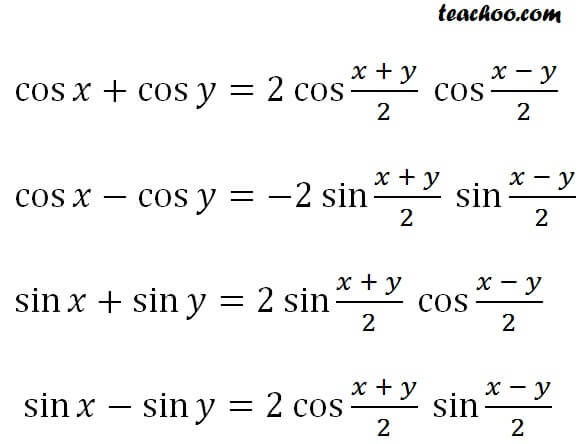Learn All Concepts of Chapter 2 Class 11 Relations and Function - FREE. Check - Trigonometry Class 11 - All Concepts

Sum to product identities are1. Chapter 3 Class 11 Trigonometric Functions
2. Concept wise
3. Cos x + cos y formula

Transcript

cos x + cos y = 2 cos (x+y)/2 cos (x -y)/2 cos x - cos y = - 2 sin (x+y)/2 sin (x -y)/2 sin x + sin y = 2 sin (x+y)/2 cos (x -y)/2 sin x - sin y = 2 cos (x+y)/2 sin (x -y)/2

Cos x + cos y formula